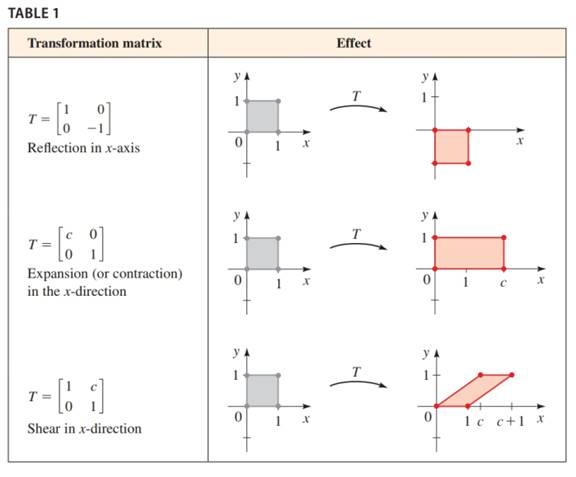Chapter 11.FOM, Problem 3P### Algebra and Trigonometry (MindTap ...

4th Edition
James Stewart + 2 others
ISBN: 9781305071742

#### Solutions

Chapter
Section### Algebra and Trigonometry (MindTap ...

4th Edition
James Stewart + 2 others
ISBN: 9781305071742
Textbook Problem

# Let T = [ 1 1.5 0 1 ] (a) What effect does T have on the gray square in the Table 1.(b) Find T − 1 .(c) What effect does T − 1 have on the gray square?(d) What happens to the square if we first apply T , then T − 1 ?To determine

(a)

To find:

The effect of T on the gray square.

Explanation

Given:

The vertices of the gray square are:

, , , and .

The matrix T=[11.501]

Approach:

Multiplication of Matrices:

If A is a m×n matrix and B is a n×p matrix, then the product AB is the m×p matrix whose ijelement is the inner product of the ith row of A and jth column of B.

Transformation matrix:

Reflection in xaxis,

T=

Expansion in xdirection,

T=[c001]

Shear in

To determine

(b)

To find:

The value of T1.

To determine

(c)

To find:

The effect of T1 on the gray square.

To determine

(d)

To find:

Effect on square when T is applied then T1.

### Still sussing out bartleby?

Check out a sample textbook solution.

See a sample solution

#### The Solution to Your Study Problems

Bartleby provides explanations to thousands of textbook problems written by our experts, many with advanced degrees!

Get Started